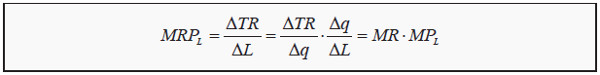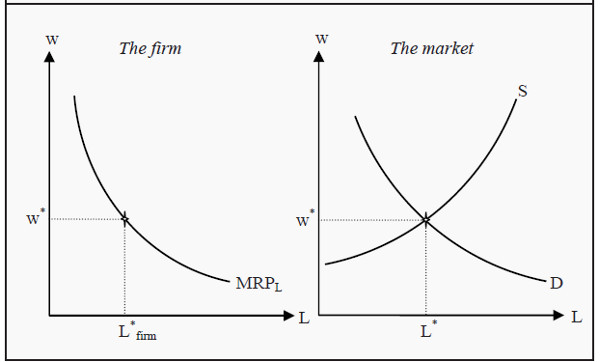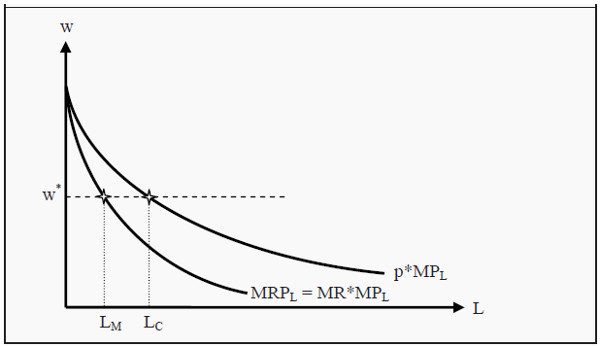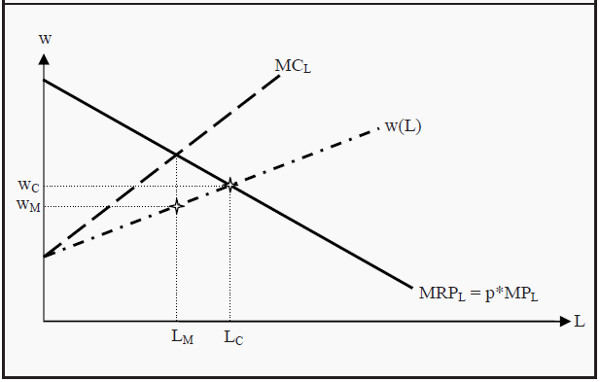# Labor

To produce goods and services, a firm uses raw materials, labor, and capital. We will now look at the market for labor. The workers sell their labor, or alternatively the sell their leisure time, for a wage, and their supply depends on their valuations of leisure and wage, respectively.

From the firm’s perspective, it buys labor as long as that gives a positive contribution to its profit. The firm’s cost of labor is the wage, and its revenue of labor is the price at which they can sell the goods. The firm will consequently hire workers until the last produced unit of the good costs as much to produce as the firm is paid for it.

This means that the structure in the output market, i.e. the market where the firm sells its goods, will also affect what the firm will be willing to pay in wages since it is in the output market that the price is set. We will study the cases when the output market is a perfectly competitive market and when it is a monopoly market.

Furthermore, the structure of the labor market also affects the outcome. We study cases in which either the firm, or the workers, or both of them are in a monopoly position or in a perfectly competitive situation.

## The Supply of Labor

We will assume that the workers prefer leisure to work and that they work for, and only for, the wage. There are 24 hours in a day, which sets an upper bound for how much labor a worker can sell. To analyze the supply of labor, it is useful to redefine the question: Instead of studying the supply of labor, we will study the demand for leisure. The supply of work will then be 24 minus the amount of leisure. Then, we can analyze the situation as in Figure 16.1.

The situation here is a variation of the analyses we performed in Section: The Individual Demand Curve. As we are studying the consumption of two goods, leisure and wage (where, again, it is useful to think of money, the wage, as “all other goods than leisure”), increases and decreases in wage will have both substitution- and income effects.

To see the similarity with the analysis in , note that one can view an increase in wage as a decrease in the price of “all other goods.” The budget line will then rotate around a fixed point at 24 (as a day has exactly 24 hours) on the X-axis and intersect the Y-axis at different points depending on the wage. Compare, for instance, to Figure 5.1. (However, note one thing: Here, the price of the other good changes (i.e. wage, not leisure), not the price of the one we are analyzing.)

In Figure 16.1, we have drawn four budget lines, corresponding to four different levels of income, w (for wage), and four indifference curves. The indifference curves, as always, indicate combinations of the two goods that the individual is indifferent between, and she strives to maximize her utility given the budget restriction. As before, the point of maximization occurs where an indifference curve just barely touches a budget line.

We have indicated four such points of tangency in the figure, and then connected them to a curve. That curve corresponds to the individual’s demand for leisure, and indirectly (if you take 24 minus her demand for leisure) to her supply of labor.

The odd thing about the supply curve for labor is that it slopes back again at high wages. Remember that the effect of a price change can be divided into a substitution effect and an income effect. At initially low wages, an increase in the wage often leads to an increase in the labor supplied.

That is due to the substitution effect dominating over the income effect. The substitution effect makes the wage more attractive relative leisure, whereas the income effect makes the individual wealthier. The increase in wealth can lead to increased consumption of both “other goods” (the wage) and of leisure.Figure 16.1: The Individual’s Supply of Labor

The higher the wage is, the more important the income effect will be, until finally, it will start to dominate over the substitution effect. If a well-paid individual has her wage increased even further, she may choose to work less than she used to. This is what makes the supply curve for labor bend backward for high wages.

## The Marginal Revenue Product of Labor

In Chapter 7, we studied the firm’s production, and we defined the production function as a function of labor, L, and capital, K, such that q = f(L,K). In the long run, both L and K are variable, in the short run only L is variable. If the firm buys one more unit (for instance, one more hour) of labor, how will that affect the profit? Remember that we defined the marginal product of labor, MPL, in Section: Average and Marginal Product. In words, MPL is how much more of the good that will be produced if we increase labor by a small amount (say, one more unit), given that everything else is held constant. When the firm decides whether to buy more labor, it first asks how large the value of the extra production is, i.e. how valuable is MPL? We can express this value mathematically asHere, MRPL, is the marginal revenue product of labor. It corresponds to how much total revenue, TR, changes because of a small increase in L. In the third step above, we have divided and multiplied by Δq in order to show that MRPL = MR*MPL. In other words, if we hire one more unit of labor, the value-added is how many additional units of the good is produced during that hour (MPL) times at which price can we sell each additional unit (MR). That value-added is called the marginal revenue product of labor.

## The Firm’s Short-Run Demand for Labor

As we have mentioned, the firm’s demand for labor depends on what the market for the firm’s output looks like, as well as on the level of competition in the labor market.

### Perfect Competition in both the Input and Output Market

In the simplest case, we have perfect competition in both the input and in the output market. The firm cannot influence the price, p, on the good, which, in turn, makes the marginal revenue equal to the price (see Section Strategy to Find the Optimal Short-Run Quantity):

MR = p

The value of the marginal product of labor is consequently MRPL = p*MPL. Furthermore, the marginal cost of labor equals the wage, MCL = w. The firm will then hire workers as long as MRPL > w, i.e. as long as the revenue is higher than the cost of hiring, and the criterion for equilibrium is

### MRP =w

Note now that this means that the MRPL curve will become the firm’s demand curve for labor. Furthermore, remember that we have the law of diminishing marginal returns. MRPL = p*MPL will therefore, eventually, start to diminish the more workers we hire (since MPL will diminish while p is constant). We will then get a downward sloping demand curve for labor, as in the left part of Figure 16.2. (Compare also to Figure 7.1.)Figure 16.2: The Firm’s Demand for Labor and the Market Equilibrium

Since we have perfect competition in the labor market, both the firm and the workers take the wage, w, as given, and as we have perfect competition in the output market as well, the firm takes p as given. The market’s demand for labor is the sum of all firms’ demand curves, and the market’s supply is the sum of all individuals’ supply curves (to the right in Figure 16.2). The individual firm will then hire workers until

MRPL = w

### Monopoly in the Output Market

We continue to assume that there are many buyers and sellers of labor, but now we assume that the good is sold in a monopoly market. The firm maximizes profit in the same way as before, i.e. it hires workers until the cost, w, is as large as the marginal revenue product, MRPL. However, since the good is now sold in a monopoly market, MR will not be equal to the price anymore. Instead,

MR (< p) will fall with increased production (see Section: Demand and Marginal Revenue). This, in turn, means that MRPL (= MR*MPL) will be steeper than in the case of perfect competition in the output market (since now both MRPL and MR are downward sloping curves). The monopolist produces a smaller quantity than a firm in a competitive market does, and therefore she will hire fewer workers.Figure 16.3: The Demand for Labor when the Output Market is a Monopoly

In Figure 16.3, we have drawn the demand curves for labor, both for a monopolist and for a firm in a competitive market. The monopolist’s demand curve is MRPL (= MR*MPL) and it will lie below the MRPL (= p*MPL) for a firm in a competitive market. The wage, w, is set in a competitive labor market and cannot be affected by either workers or firms. However, the firm hires fewer workers, LM, than it would in a competitive market, LC. A monopoly in the output market will consequently create inefficiencies in both the market for goods and in the labor market.

### Monopsony in the Input Market

Monopsony (mono = one; opsonia = buy) means that there is only one buyer. In the labor market, this means that there is only one buyer of labor. In countries where the government operates the health care system, it is, in effect, a monopsonist on, for instance, the market for nurses. The analysis of monopsonies parallels one of the monopolies.

Suppose, again, that there is perfect competition in the output market and that that there are many sellers of labor. However, there is only one buyer of labor. If the monopsonist increases the wage, she must do so for all workers, even the ones she has already hired. Thereby, her marginal cost of hiring one additional unit of labor is higher than the wage to the last worker. (Compare to the reasoning regarding MR for a monopolist)

Since the monopsonist is the only buyer in the market, she faces the whole supply of the market. (Compare to the monopolist, who faces the whole demand of the market.) Her marginal cost of labor, MCL, will therefore have a steeper slope than the supply curve (see Figure 16.4).Figure 16.4: Monopsony

As in the other cases, the monopsonist hires workers as long as she gets higher revenues from doing so than she has to pay in wages. Now, since MC is not equal to the wage anymore, she does so as long as MRPL > MCL. In equilibrium

MRP L =MC L

Note now that, in the previous sections, Perfect Competition in both the Input and Output Market and Monopoly in the Output Market, we had that MCL = w. Now, however, we have that MCL > w. In Figure 16.4, the monopsonist hires LM workers and pays a wage of wM. Comparing to the case when we have competition in the labor market, we see that the wage in a monopsony, wM, is lower and that the firm hires fewer workers, LM < LC. This parallels the results from the monopoly market, where the monopolist produced a smaller quantity than a perfectly competitive market did, and charged a higher price per unit.

### Bilateral Monopoly

A bilateral monopoly is a situation in which there is only one buyer and one seller. Both parties will then have market power, and the outcome depends largely on negotiations, the business cycle, etc. This resembles the situation in some countries where there are centralized negotiations between unions representing the workers and other organizations representing the employers.